# Methods and formulas for variance components in Fit Mixed Effects Model

Select the method or formula of your choice.

## Variance components

The variance components require an iterative solution to estimate the parameter θi. Once you have the parameter, the variance components have explicit solutions. The formula for the variance component for error is:

where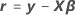The following are the variance components for the random effect terms:

For details on the estimation of θi, see .

For further details on the notation, go to the Methods section.

### References

1. Hemmerle, W. and Hartley, H. (1973). Computing Maximum Likelihood Estimates for the Mixed A.O.V. Model using the W transformation. Technometrics, 15(4):819–831.

## Standard errors of variance components

To estimate the standard errors of the variance components, Minitab begins with the observed Fisher information matrix. The matrix has c + 1 rows and columns. The variable c is the number of random effect terms in the model and 1 represents the variance for the error term. For i = 1, …, c and j = 1, …, c the following is the formula for the ijth component of the observed Fisher information matrix: where
The following formula is the component of the last row and the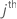column, j = 1, …, c: where

This component is also the value of the last column and therow by the symmetry property of the variance-covariance matrix.

The following formula is the component of the last row and the last column:

The asymptotic variance-covariance matrix for the variance components estimates is twice the inverse of the observed Fisher information matrix. The estimates of the standard errors are the square roots of the diagonal elements of the variance-covariance matrix. The first c diagonal elements are for the variance components of the random effect terms. The last diagonal element is for the error variance component.

### Notation

TermDescription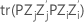the trace of matrix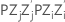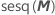the sum of squares of all the elements in the matrix M

For further details on the notation, go to the Methods section.

## Confidence intervals for variance components

Minitab uses the delta method to construct Wald-type confidence limits for the natural log of the variance components, then exponentiates the confidence intervals to get the confidence intervals for the variance components. The formulas for the variance component for error have the same form.

### Notation

TermDescription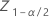the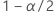quantile from the standard normal distribution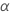1 − confidence level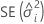the standard error of thevariance component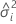the variance component for therandom effect term

## Z-value and p-value

The null and alternative hypotheses for the test are: The hypotheses for the error variance are the same.
The test statistic assumes a standard normal distribution:
The p-value is the upper tail probability from the standard normal distribution under the null hypothesis:

### Notation

TermDescription
Zthe value of the inverse cumulative distribution function for the standard normal distribution

## Variance-covariance matrix

The asymptotic variance-covariance matrix is the inverse of the observed Fisher information matrix. The matrix has c + 1 rows and columns. The variable c is the number of random effect terms in the model and 1 represents the variance for the error term. For i = 1, …, c and j = 1, …, c the following is the formula for the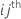component of the observed Fisher information matrix: where
The following formula is the component of the last row and thecolumn, j = 1, …, c: where

This component is also the value of the last column and therow by the symmetry property of the variance-covariance matrix.

The following formula is the component of the last row and the last column:

### Notation

TermDescriptionthe trace of matrixFor further details on the notation, go to the Methods section.

By using this site you agree to the use of cookies for analytics and personalized content.  Read our policy